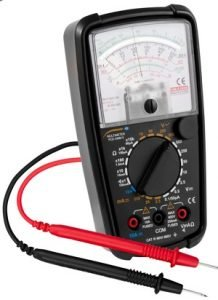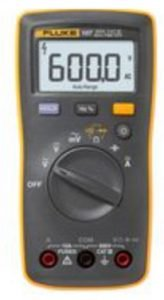# Basics of electricity

Voltmeter: A voltmeter is an instrument used for measuring an electrical potential difference between two points in an electric circuit. Analog voltmeters move a pointer across a scale in proportion to the voltage of the circuit; digital voltmeters give a numerical display of voltage by use of an analog to digital converter. Potential difference: Voltage, electrical potential difference, electric tension or electric pressure (denoted ∆V and measured in units of electric potential: volts, or joules per coulomb) is the electric potential difference between two points or the difference in electric potential energy of a unit charge transported between two points. Electric field: An electric field is generated by electrically charged particles and time-varying magnetic fields. The electric field describes the electric force experienced by a motionless electrically charged test particle at any point in space relative to the source(s) of the field. The concept of an electric field was introduced by Michael Faraday.

Figure 1 displays an analogue voltmeter model PCE-AMM5. Figure  2 displays a digital multimeter model Fluke-107 ESP  10A, 600V, 40MOHMFigure 1